# For goodness of fit's sake

No, this is not a recap from the O.J. Simpson trial, but it is a question we face whenever we propose a model for our data: does the model fit, and does it explain the data variation properly?

In a time series modeling process, we seek a quantitative measure of the discrepancy (or the goodness of fit) between the observed values and the values expected under the model in question. The discrepancy measure is crucial for two important applications: (1) finding the optimal values of the model’s parameters, and (2) comparing competing models in an attempt to nail the best one. We believe that the model with the best fit should give us superior predictions for future values.

A few questions might spring to mind: What are the goodness-of-fit functions? How are they different from each other? Which one should I use? How are they related to the normality test?

In this tutorial, we’ll discuss the different goodness of fit functions through an example of the monthly average of ozone levels in Los Angeles between 1955 and 1972. This data set was used by Box, Jenkins, and Riesel in their time-series textbook – Time Series Forecast and Control, published in 1976.

## Background

We’ll start with the likelihood function, and then cover derivative measures (e.g. AIC, BIC, HQC, etc.)

The likelihood function is defined as a function of the model parameters $(\theta)$ of the statistical model. The likelihood of a set of parameter values given some observed outcomes ($\mathcal{L} (\theta | x)$) is equal to the probability of those observed outcomes given those parameter values $(f_\theta (x))$.

$$\mathrm{L}(\theta |x)=f_\theta (x)$$

Where:

• $f_\theta=$probability mass (density) function

Assuming {${x_t}$} consists of independent and identically distributed (i.i.d) observations, the likelihood function of a sample set is expressed as follows: $$\mathcal{L}(\theta |x_1,x_2,...,x_T)=f_\theta(x_1,x_2,...,x_T)=f_\theta(x_1)f_\theta.(x_2).f_\theta(x_3)...f_\theta(x_T)=\prod_{t=1}^{T}f_\theta(x_t)$$

To overcome the diminishing value of $\mathcal{L}(\theta |x_1,x_2,...,x_T)$ as the sample size increases and to simplify its calculation, we take the natural logarithm of the likelihood function.

$$LLF(\theta | x_1,x_2,...,x_T)=\ln(\mathcal{L}(\theta | x_1, x_2,...x_T))=\sum_{t=1}^{T}\ln(f_\theta(x_t))$$

Example 1: Gaussian distribution

$$LLF(\mu,\sigma |x_1,x_2,...,x_T )=\sum_{t=1}^{T}\ln(\frac{e^\frac{(x_t-\mu )^2}{2\sigma^2 }}{\sqrt{2\pi\sigma }})=\frac{-T\ln(2\pi\sigma^2 )}{2}-\sum_{t=1}^{T}\frac{(x_t-\mu )^2}{2\sigma ^2}$$

$$LLF(\mu,\sigma |x_1,x_2,...,x_T )=\frac{-T\ln(2\pi\sigma ^2)-(T-1)\left ( \frac{ \hat{\sigma}}{\sigma} \right )^2}{2}$$

$$LLF(\mu =0,\sigma^2=1 |x_1,x_2,...,x_T )=- \frac{1}{2} (T\ln (2\pi)+(T-1){\sigma^*}^2)$$

Where:

• $\sigma^* =$ the un-biased estimate of the standard deviation
• $\sigma=$ the distribution standard deviation
• $\mu=$ the sample data mean or average

Note:

The log-likelihood function is linearly related to the sample data variance; as the sample data variance increases (fit is worse), the log-likelihood decreases, and vice versa.

## Models comparison

Typically, we use the LLF in searching for the optimal values of the coefficients of a model using one sample data set.

To compare the goodness of fit between models, we encounter two main challenges:

1. The number of free parameters $(k)$ in each model is different. Using LLF as it stands now, it is possible the LLF will give greater weight to complex models, as they can (theoretically) over-fit the sample data.
2. Due to the different lag orders in each model, the number of remaining non-missing observations $(N)$ can differ between models, assuming we use the same sample data with all models.

We use two distinct measures to address these issues:

## Akaike’s Information Criterion (AIC)

$$AIC=-2\times LLF+\frac{2\times N\times k}{N-k-1}$$ $$AICc=AIC+\frac{2k(k+1)}{N-k-1}=-2\times LLF+\frac{2\times N\times k}{N-k-1}$$

The original definition (AIC) adds a linear penalty term for the number of free parameters, but the AICc adds a second term to factor into the sample size, making it more suitable for smaller sample sizes.

## Bayesian (Schwarz) Information Criterion (BIC/SIC or SBC)

$$BIC=-2\times LLF+k\ln(N)$$

As in AIC, the BIC penalizes the model complexity $(k)$. Given any two estimated models, the model with the lower value of BIC is preferred.

The BIC generally penalizes free parameters more strongly than does the AIC, though it depends on the size of n and the relative magnitude of n and k.

Ozone levels in downtown Los Angeles

In this tutorial, we’ll use the monthly average of the hourly ozone levels in LA between Jan 1955 and Dec 1972.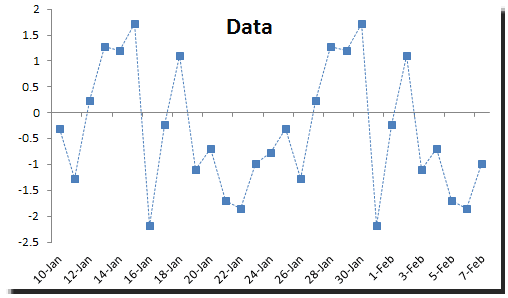The underlying process exhibits seasonality around a 12-month period, but it appears to decay over time. There are two important facts to consider about this example:

1. In 1960, two major events occurred, which might have reduced ozone levels: (1) the Golden State Freeway was opened, and (2) a new rule (rule 63) was passed to reduce the allowable proportion of hydrocarbons in locally-sold gasoline.
2. In 1966, the regulation was adopted requiring engine design changes that were expected to reduce the production of ozone in new cars.

The underlying process had undergone major changes throughout the sample data period. For forecasting purposes, we will exclude the observations between 1955 and 1966. For this tutorial, we assume we did not know about those events and simply ignore them.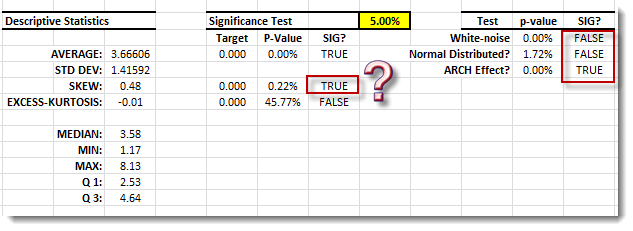The summary statistics suggest the following: (1) serial correlation, (2) arch effect, and (3) significant skew.

Next, let’s examine the ACF and PACF plot (correlogram).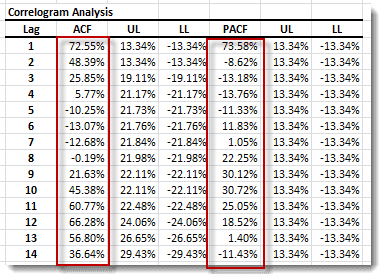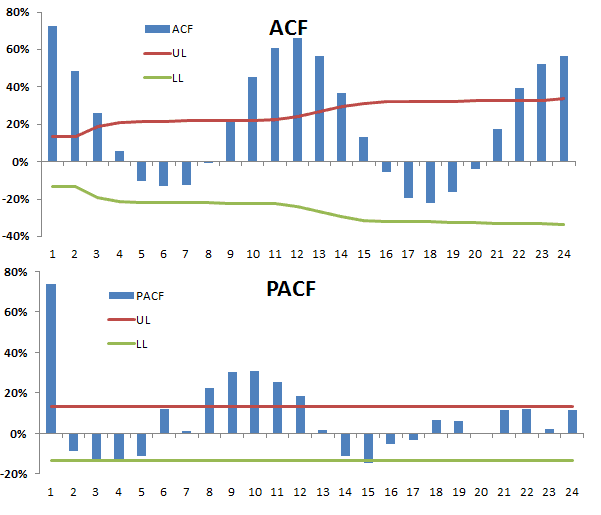The correlogram (ACF and PACF) appears similar to an Airline type of model with a 12-month period of seasonality. We went ahead and constructed the model, then calibrated its parameter values using the sample data.

$$(1-L)(1-L^{12})x_t=\mu +(1-\theta L)(1-\Theta L^{12})a_t$$ $$a_t=\sigma \times\varepsilon _t$$ $$\varepsilon_t\sim i.i.d\sim N(0,1)$$

Note:

The calibration process is a simple maximization problem with the LLF as the utility function and the model’s validity function as the only constraint. We can also use AIC or BIC as the utility function instead of the LLF, but we have to search for parameter values that minimize the utility.

Let’s now compute the standardized residuals and determine the different goodness-of-fit measures: LLF, AIC, and BIC.

1. Compute the residuals

1. The Hard way: Using the AIRLINE_MEAN function, get the estimated model’s values and subtract those from the observed values to get the raw residuals. Subtract the residuals’ mean from the raw residuals and divide by standard deviation to get the standardized residuals.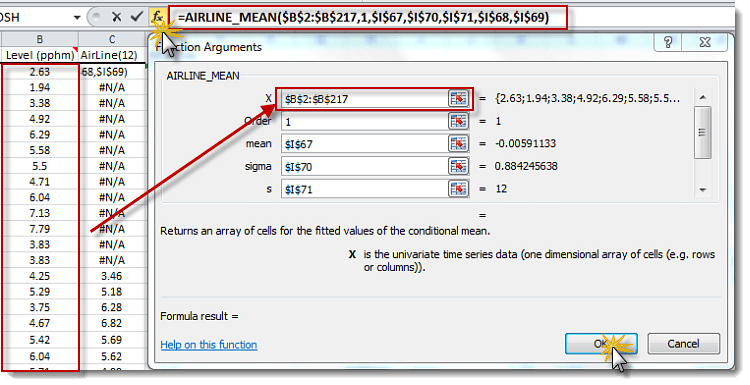2. The easy way: Using the AIRLINE_RESID function will yield an array of the standardized residuals of the model.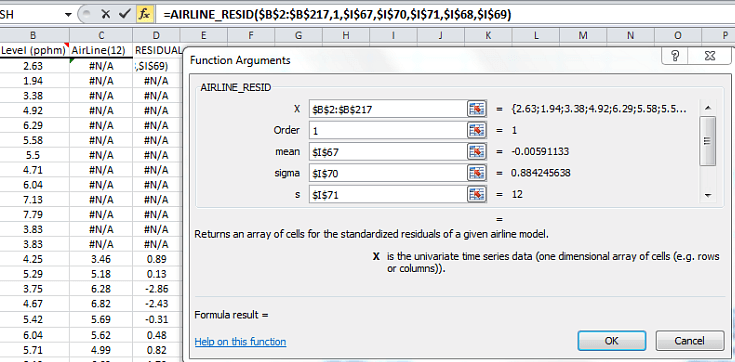Let’s plot the distribution (and QQ-Plot) of the standardized residuals.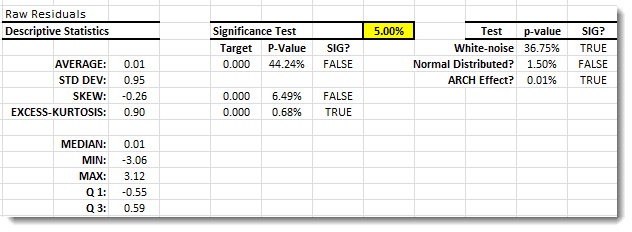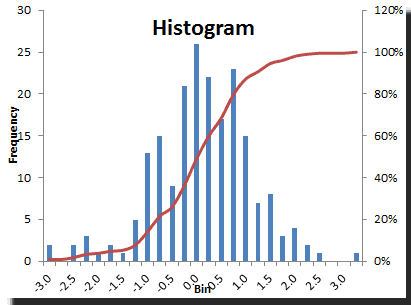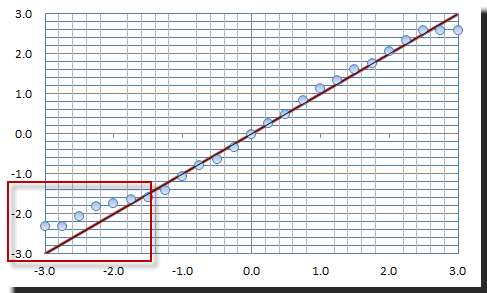2. Compute the log-likelihood for the standardized residuals

Now, let’s compute the log-likelihood function. We can do that either by computing the log of the mass function at each point and then adding them together, or simply by using this formula:

$$LLF=-\frac{1}{2}(N\ln(2\pi)+(N-1)\hat{\sigma }^2)$$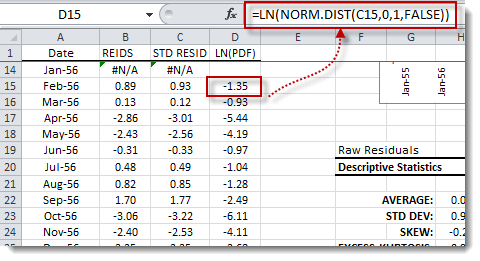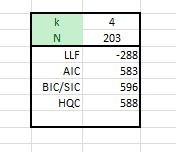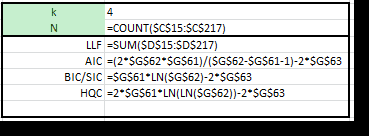Note:

• The number of non-missing points is 203 (i.e. 216 – 13). We have lost 13 points.
• LLF is not identical to the one we had earlier (LLF = -265) in the Airline model table. The AIRLINE_LLF uses Whittle’s approximation to compute the LLF function, which is relatively close and efficient for large sample data.
• The AIC and BIC values are relatively close, but the BIC penalizes more than AIC.

## Conclusion

The log-likelihood function offers an intuitive way to think about a model’s fit with a sample data set, but it lacks any consideration for the model’s complexity or sample size. Thus, it is not appropriate for comparing models of different orders.

The Akaike and Bayesian information criteria fill the gap that LLF leaves when it comes to comparing models. They both offer penalty terms for the number of free parameters and the number of non-missing observations. Furthermore, in practice, the BIC is more often used than the AIC, especially in model identification and selection processes.

To compare models, please note that we need to use the same sample data with all models. We can’t use AIC or BIC to compare two models, each computed using a different data set.